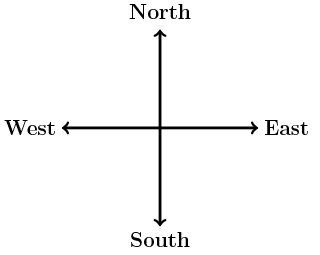# POLQ 1 | Lesson 4 | Making Connections (Applying Understanding of Polynomials)# Applying Understanding of Polynomials

The diagram below represents the Jane’s spring garden plan.  Although you might never see a garden with these measures (in terms of a variable), you will use your knowledge of the computation and simplification of polynomials to answer the questions below.1. Write and simplify a polynomial expression that represents the south side of the field.
2. Write polynomial expressions that represent: the perimeter of the carrot patch and the perimeter of the lettuce patch.
3. Find the area of the same two patches mentioned in question two.
4. Assume that $$x=4$$, determine the perimeter and area of the carrots patch. Dimensions are in feet.
5. Jane would like to plant three additional patches of produce. Using factoring, determine possible dimensions of each of the patches below:

a. The area of the strawberry patch is $$16x^2+4x$$.

b. The area of the cucumber patch is $$x^2-4x-21$$.

c. The area of the tomato patch is $$x^2-36$$.

1. Jane is adding a zucchini patch that has dimensions that are half those of the watermelon patch. Find and simplify polynomials that would represent the dimensions of the zucchini patch.

Check solutions here.